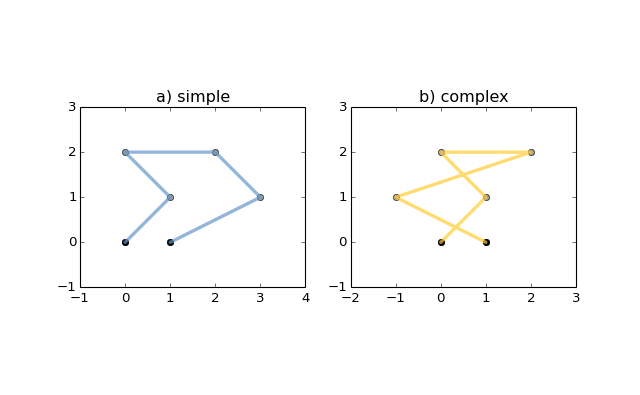# TensorFlow 图神经网络框架tf_geometric荐国

GPL

2020-01-22
CrawlScript

tf_geometric是一个高效且友好的图神经网络框架，同时支持TensorFlow 1.x和2.x。

tf_geometric使用消息机制来实现图神经网络，这会比基于普通矩阵实现的版本更为高效且比基于稀疏矩阵实现的版本更为友好。另外，框架为复杂的图神经网络操作提供了简单优雅的API。下面的示例构建了一个图，并对其执行了多头图注意力网络（GAT）：

```# coding=utf-8
import numpy as np
import tf_geometric as tfg
import tensorflow as tf

graph = tfg.Graph(
x=np.random.randn(5, 20),  # 5 nodes, 20 features,
edge_index=[[0, 0, 1, 3],
[1, 2, 2, 1]]  # 4 undirected edges
)

print("Graph Desc: \n", graph)

graph.convert_edge_to_directed()  # pre-process edges
print("Processed Graph Desc: \n", graph)
print("Processed Edge Index:\n", graph.edge_index)

# Multi-head Graph Attention Network (GAT)
output = gat_layer([graph.x, graph.edge_index])
print("Output of GAT: \n", output)```

Output:

``````Graph Desc:
Graph Shape: x => (5, 20)	edge_index => (2, 4)	y => None

Processed Graph Desc:
Graph Shape: x => (5, 20)	edge_index => (2, 8)	y => None

Processed Edge Index:
[[0 0 1 1 1 2 2 3]
[1 2 0 2 3 0 1 1]]

Output of GAT:
tf.Tensor(
[[0.22443159 0.         0.58263206 0.32468423]
[0.29810357 0.         0.19403605 0.35630274]
[0.18071976 0.         0.58263206 0.32468423]
[0.36123228 0.         0.88897204 0.450244  ]
[0.         0.         0.8013462  0.        ]], shape=(5, 4), dtype=float32)
``````

## 面向对象和函数式API

```# coding=utf-8
import os

# Enable GPU 0
os.environ["CUDA_VISIBLE_DEVICES"] = "0"

import tf_geometric as tfg
import tensorflow as tf
import numpy as np
from tf_geometric.utils.graph_utils import convert_edge_to_directed

# ==================================== Graph Data Structure ====================================
# In tf_geometric, graph data can be either individual Tensors or Graph objects
# A graph usually consists of x(node features), edge_index and edge_weight(optional)

# Node Features => (num_nodes, num_features)
x = np.random.randn(5, 20).astype(np.float32) # 5 nodes, 20 features

# Edge Index => (2, num_edges)
# Each column of edge_index (u, v) represents an directed edge from u to v.
# Note that it does not cover the edge from v to u. You should provide (v, u) to cover it.
# This is not convenient for users.
# Thus, we allow users to provide edge_index in undirected form and convert it later.
# That is, we can only provide (u, v) and convert it to (u, v) and (v, u) with `convert_edge_to_directed` method.
edge_index = np.array([
[0, 0, 1, 3],
[1, 2, 2, 1]
])

# Edge Weight => (num_edges)
edge_weight = np.array([0.9, 0.8, 0.1, 0.2]).astype(np.float32)

# Make the edge_index directed such that we can use it as the input of GCN
edge_index, edge_weight = convert_edge_to_directed(edge_index, edge_weight=edge_weight)

# We can convert these numpy array as TensorFlow Tensors and pass them to gnn functions
outputs = tfg.nn.gcn(
tf.Variable(x),
tf.constant(edge_index),
tf.constant(edge_weight),
tf.Variable(tf.random.truncated_normal([20, 2])) # GCN Weight
)
print(outputs)

# Usually, we use a graph object to manager these information
# edge_weight is optional, we can set it to None if you don't need it
graph = tfg.Graph(x=x, edge_index=edge_index, edge_weight=edge_weight)

# You can easily convert these numpy arrays as Tensors with the Graph Object API
graph.convert_data_to_tensor()

# Then, we can use them without too many manual conversion
outputs = tfg.nn.gcn(
graph.x,
graph.edge_index,
graph.edge_weight,
tf.Variable(tf.random.truncated_normal([20, 2])),  # GCN Weight
cache=graph.cache  # GCN use caches to avoid re-computing of the normed edge information
)
print(outputs)

# For algorithms that deal with batches of graphs, we can pack a batch of graph into a BatchGraph object
# Batch graph wrap a batch of graphs into a single graph, where each nodes has an unique index and a graph index.
# The node_graph_index is the index of the corresponding graph for each node in the batch.
# The edge_graph_index is the index of the corresponding edge for each node in the batch.
batch_graph = tfg.BatchGraph.from_graphs([graph, graph, graph, graph])

# Graph Pooling algorithms often rely on such batch data structure
# Most of them accept a BatchGraph's data as input and output a feature vector for each graph in the batch
outputs = tfg.nn.mean_pooling(batch_graph.x, batch_graph.node_graph_index, num_graphs=batch_graph.num_graphs)
print(outputs)

# We can reversely split a BatchGraph object into Graphs objects
graphs = batch_graph.to_graphs()

# ==================================== Built-in Datasets ====================================
# all graph data are in numpy format

# we can convert them into tensorflow format
test_data = [graph.convert_data_to_tensor() for graph in test_data]

# ==================================== Basic OOP API ====================================
# OOP Style GCN (Graph Convolutional Network)
gcn_layer = tfg.layers.GCN(units=20, activation=tf.nn.relu)

for graph in test_data:
# Cache can speed-up GCN by caching the normed edge information
outputs = gcn_layer([graph.x, graph.edge_index, graph.edge_weight], cache=graph.cache)
print(outputs)

# OOP Style GAT (Multi-head Graph Attention Network)
for graph in test_data:
outputs = gat_layer([graph.x, graph.edge_index])
print(outputs)

# ==================================== Basic Functional API ====================================
# Functional Style GCN
# Functional API is more flexible for advanced algorithms
# You can pass both data and parameters to functional APIs

gcn_w = tf.Variable(tf.random.truncated_normal([test_data.num_features, 20]))
for graph in test_data:
outputs = tfg.nn.gcn(graph.x, edge_index, edge_weight, gcn_w, activation=tf.nn.relu)
print(outputs)

# ==================================== Advanced OOP API ====================================
# All APIs are implemented with Map-Reduce Style
# This is a gcn without weight normalization and transformation.
# Create your own GNN Layer by subclassing the MapReduceGNN class
class NaiveGCN(tfg.layers.MapReduceGNN):

def map(self, repeated_x, neighbor_x, edge_weight=None):
return tfg.nn.identity_mapper(repeated_x, neighbor_x, edge_weight)

def reduce(self, neighbor_msg, node_index, num_nodes=None):
return tfg.nn.sum_reducer(neighbor_msg, node_index, num_nodes)

def update(self, x, reduced_neighbor_msg):
return tfg.nn.sum_updater(x, reduced_neighbor_msg)

naive_gcn = NaiveGCN()

for graph in test_data:
print(naive_gcn([graph.x, graph.edge_index, graph.edge_weight]))

# ==================================== Advanced Functional API ====================================
# All APIs are implemented with Map-Reduce Style
# This is a gcn without without weight normalization and transformation
# Just pass the mapper/reducer/updater functions to the Functional API

for graph in test_data:
outputs = tfg.nn.aggregate_neighbors(
x=graph.x,
edge_index=graph.edge_index,
edge_weight=graph.edge_weight,
mapper=tfg.nn.identity_mapper,
reducer=tfg.nn.sum_reducer,
updater=tfg.nn.sum_updater
)
print(outputs)
```

## 示例

• Graph Convolutional Network (GCN)
• Multi-head Graph Attention Network (GAT)

### 评论(0)#### 暂无评论#### 暂无资讯#### 暂无问答

CGAL Python Bindings

CGAL Python Bindings WARNING: This project(http://cgal-python.gforge.inria.fr/) is no longer maintained. Please try CGAL-bindings that provides similar functionalities. The goal...

2016/01/12 11:15
460
1
Shapely地理空间几何库，使用手册（英）

2016/01/12 14:35
8.4K
1TensorFlow 机器学习秘籍中文第二版（初稿）

TensorFlow 入门 介绍 TensorFlow 如何工作 声明变量和张量 使用占位符和变量 使用矩阵 声明操作符 实现激活函数 使用数据源 其他资源 TensorFlow 的方式 介绍 计算图中的操作 对嵌套操作分层...

2019/09/18 00:19
191
0

2019/09/28 23:53
43
0

TensorFlow 101 什么是 TensorFlow？ TensorFlow 核心 代码预热 - Hello TensorFlow 张量 常量 操作 占位符 从 Python 对象创建张量 变量 从库函数生成的张量 使用相同的值填充张量元素 用序...

2019/09/16 14:14
66
0